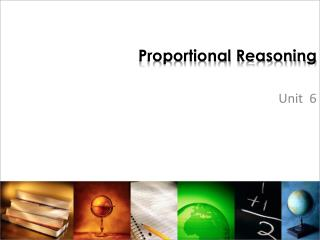DownloadDownload PresentationProportional Reasoning

# Proportional Reasoning

Télécharger la présentation## Proportional Reasoning

- - - - - - - - - - - - - - - - - - - - - - - - - - - E N D - - - - - - - - - - - - - - - - - - - - - - - - - - -
##### Presentation Transcript

1. Proportional Reasoning Unit 6

2. Writing Ratios • 12 dogs to 6 cats • 12/6 • Reduced to 2/1 • 2:1 • 2 to 1

3. Writing ratios • 8 boys to 12 girls • 8/12 • Reduced to 2/3 • 2:3 • 2 to 3

4. Finding Equivalent Ratios • 2/3 • 4/6 • 8/12 • 20/30 • ________ • _________

5. Finding Equivalent Ratios • 4/5 • 12/15 • 20/25 • 40/50 • __________ • __________

6. Proportion or not? • To determine if a ratio is a proportion or not, cross multiply. • If the products are equal, the ratio is a proportion • If the products are not equal, the ratio is NOT a proportion

7. Proportion or not? • 2/3 ; 18/27 • 5/7 ; 20/27

8. Proportion or not? • 12/15 ; 8/10 • 6/10 ; 9/15

9. Solving Proportions • To solve a proportion, cross multiply the way you have two numbers. • Then divide by the number that is across from the variable. 8 = X 1 4 21

10. Solving Proportions 6 = z_ 8 48

11. Solving Proportions 8 = 16 17 x

12. Solving Proportions 14 = 8.4 w 4.5

13. Finding Unit Rate • You always want the second number to be 1. • Divide both numbers by the second number. • Example: 330 miles in 6 hours • We want to know how many miles in 1 hour. • Divide both numbers by 6 so the second number will be a 1. • 55 miles in 1 hour (55 mi/hr)

14. Finding Unit Rate • 156 students in 6 classes

15. Finding Unit Rate • 424 Calories in 3 servings

16. Finding Unit Rate • \$231 for 3 game tickets • **always put money on top if there is money in the problem.

17. Finding Unit Rate • 5 tablespoons in 4 quarts

18. Finding Unit Rates • A farmer sells 8 ears of corn for \$3.50. How much is this per ear?

19. Missing Sides in Similar Figures 15 cm 10 cm x cm 8 cm 6 cm 9 cm

20. Missing Sides in Similar Figures 4 in. x in. 20 in. 20 in.

21. Missing Sides in Similar Figures 5 cm. 10 cm. 8 cm. 16 cm. x cm. 20 cm.

22. Missing Sides in Similar Figures 3 cm. 4 cm. 6 cm. x cm.

23. Indirect measurement • Draw a picture if there isn’t one already. • Write a proportion • Solve by cross multiplying and dividing.

24. Indirect Measurement • A flagpole is 30 feet high and a mailbox is 3.5 feet high. The mailbox casts a shadow that is 5.25 feet long. How long is the flagpole’s shadow at the same time of the day? 3.5 ft. 30 ft. 5.25 ft. x ft.

25. Indirect Measurement • A tree house casts a shadow of 18 feet while Jenny casts a shadow 9 feet. If Jenny is 5 feet tall, how tall is the tree house? 5 ft. x ft. 9 ft. 18 ft.